Test: Mathematical Logic- 1

# Test: Mathematical Logic- 1

Test Description

## 10 Questions MCQ Test GATE Electrical Engineering (EE) 2023 Mock Test Series | Test: Mathematical Logic- 1

Test: Mathematical Logic- 1 for Computer Science Engineering (CSE) 2023 is part of GATE Electrical Engineering (EE) 2023 Mock Test Series preparation. The Test: Mathematical Logic- 1 questions and answers have been prepared according to the Computer Science Engineering (CSE) exam syllabus.The Test: Mathematical Logic- 1 MCQs are made for Computer Science Engineering (CSE) 2023 Exam. Find important definitions, questions, notes, meanings, examples, exercises, MCQs and online tests for Test: Mathematical Logic- 1 below.
Solutions of Test: Mathematical Logic- 1 questions in English are available as part of our GATE Electrical Engineering (EE) 2023 Mock Test Series for Computer Science Engineering (CSE) & Test: Mathematical Logic- 1 solutions in Hindi for GATE Electrical Engineering (EE) 2023 Mock Test Series course. Download more important topics, notes, lectures and mock test series for Computer Science Engineering (CSE) Exam by signing up for free. Attempt Test: Mathematical Logic- 1 | 10 questions in 30 minutes | Mock test for Computer Science Engineering (CSE) preparation | Free important questions MCQ to study GATE Electrical Engineering (EE) 2023 Mock Test Series for Computer Science Engineering (CSE) Exam | Download free PDF with solutions
 1 Crore+ students have signed up on EduRev. Have you?
Test: Mathematical Logic- 1 - Question 1

### Which of the following is/are tautology:​

Detailed Solution for Test: Mathematical Logic- 1 - Question 1

(a) (a v b)→(b ∧ c)
= (a + b)'+ bc
= a' b' + bc
Therefore, ((a v b) → (b ∧ c)) is contingency and not tautology.
(b) (a ∧ b) →(b v c)
= ab → b + c
= (ab)' + b + c
= a' + b' + b + c
= a' + 1 + c
= 1
So ((a ∧ b) →(b v c)) is tautology,
(c) (a v b)→ (b → c)
= (a + b) → (b' + c)
= (a+ b)' + b' + c
= a' b' + b' + c
= b' + c
So ((a v b) → (b → c)) is contingency but not tautology.
(d) (a → b) → (b→ c)
= (a' + b) → (b' + c)
= (a' + b) + b' + c
= ab' + b' + c
= b' + c
Therefore; ((a → b) → (b → c)} is contingency but not tautology.

Test: Mathematical Logic- 1 - Question 2

### ​If the proposition¬ p ⇒ q is true, then the truth value of the proposition ¬ p v (p ⇒ q), where ¬ is negation, 'v' is inclusive or and '⇒’ is implication, is

Detailed Solution for Test: Mathematical Logic- 1 - Question 2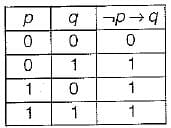Now since ¬ p → q is given true, we reduce the truth table as follows:In the reduced truth table we need to find the truth value of ¬ pv(p→q} = p' +(p → q)
≡ p' + p' + q ≡ p' + q
The truth value of p' + q in the reduced truth table is given below: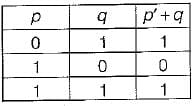Since in the reduced truth table also, the given expression is sometimes true and sometimes false, therefore the truth value of proposition ¬p v (p → q) can not be determined.

Test: Mathematical Logic- 1 - Question 3

### Let a, b, c, d be propositions. Assume that the equivalence a ⇔ ( bv ¬ b) and b ⇔ c hold. Then the truth-value of the formula (a ∧ b) → (a ∧ c) v d is always

Detailed Solution for Test: Mathematical Logic- 1 - Question 3

a ⇔ (b v ¬ b )
a ⇔ 4 True
So a is true, i.e. a = 1
b ⇔ c holds. So b = c
Now the given expression is
(a ∧ b) → ((a ∧ c) v d) ≡ (a - b ) → ((a . c) + d)
Putting a = 1 in above expression we get
1 • b → ((1 • c) + d)
≡ b→ c + d
≡ b' + c+ d
Now putting b = c in above expression we get
≡ c' + c+ d=1 + d=1
So the expression is always true.

Test: Mathematical Logic- 1 - Question 4

The following propositional statement is
(P → (Q v R)) → (( P ∧ Q) → R)

Detailed Solution for Test: Mathematical Logic- 1 - Question 4

(P → (Q v R)) → ((P ∧ Q) → R)
≡ (P→ Q+ R) → (PQ → R)
≡ [P' + Q + R] → [(PQ)' + R]
≡ [P' + Q + R] → [P' + Q' + R]
≡ (P' + Q + R)' + P' + Q' + R
≡ PQ' R' + P' + Q' + R
≡ Q' + Q' PR' + P' + R
≡ Q' + P' + R (by absorption law)
Which is a contingency (i.e. satisfiable but not valid).

Test: Mathematical Logic- 1 - Question 5

A logical binary relation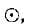is defined as follows: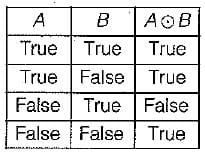Let ~ be the unary negation (NOT) operator, with higher precedence, thanWhich one of the following is equivalent to A ∧ B?

Detailed Solution for Test: Mathematical Logic- 1 - Question 5

By using min terms we can define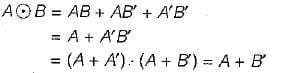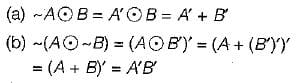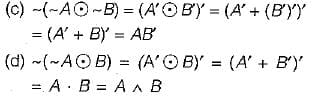∴ Only, choice (d) = A ∧ B
[Note: This problem can also be done by constructing truth table for each choice and - comparing with truth table for A ∧ B]

Test: Mathematical Logic- 1 - Question 6

Which of the following is TRUE about formulae in Conjunctive Normal Form?

Detailed Solution for Test: Mathematical Logic- 1 - Question 6

In conjunction normal form, for any particular assignment of truth values, all except one clause will always evaluate to true. So, the proportion of clauses which evaluate to true to the total number of clauses is equal to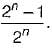Now putting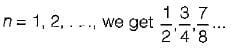All of these proportions are ≥ 1/2 and so choice (a) atleast half of the clauses evaluate to true, is. the correct answer.

Test: Mathematical Logic- 1 - Question 7

The binary operation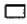is defined as follows: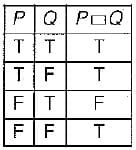Which one of the following is equivalent to P v Q?

Detailed Solution for Test: Mathematical Logic- 1 - Question 7

The given table can be converted into boolean function by adding mrnterms corresponding to true rows.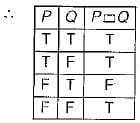Since there is only one false in the above truth table, we can represent the function PQ more efficiently, in conjunctive normal form.
Translates PQ = P + Q' (the max -term corresponding to the third row, where the function is false).
Now, we can easily translate the choices into boolean algebra as follows: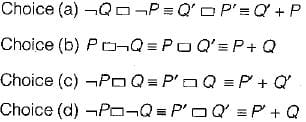As we can clearly see only choice (b)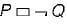is equivalent to P+ Q.

Test: Mathematical Logic- 1 - Question 8

Consider the following well-formed formulae: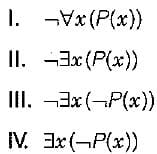Which of the above are equivalent?

Detailed Solution for Test: Mathematical Logic- 1 - Question 8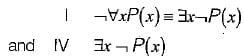Clearly, choices I and IV are equivalent.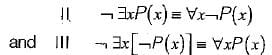Clearly II and III are not equivalent to each other or to I and IV.

Test: Mathematical Logic- 1 - Question 9

Which one of the following is NOT logically e quivalent to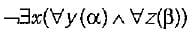Test: Mathematical Logic- 1 - Question 10

The CORRECT formula for the sentence, "not all rainy days are cold” is

Detailed Solution for Test: Mathematical Logic- 1 - Question 10

In other words it says ``Some rainy days are not cold"

Given statement is
¬∀d[R(d)→C(d)]

≡¬∀d[¬R(d)∨C(d)
≡∃d[R(d)∧¬C(d)]

## GATE Electrical Engineering (EE) 2023 Mock Test Series

21 docs|273 tests
 Use Code STAYHOME200 and get INR 200 additional OFF Use Coupon Code
Information about Test: Mathematical Logic- 1 Page
In this test you can find the Exam questions for Test: Mathematical Logic- 1 solved & explained in the simplest way possible. Besides giving Questions and answers for Test: Mathematical Logic- 1, EduRev gives you an ample number of Online tests for practice

## GATE Electrical Engineering (EE) 2023 Mock Test Series

21 docs|273 tests